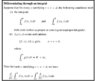# Determine the range of a function using parameter differentiation

• schniefen

#### schniefen

Homework Statement
Determine the range of ##f(x)## for ##x>0## using differentiation under the integral sign.
Relevant Equations
##f(x)=\int_x^{2x} (\frac{e^{-t^2x}}{t}) \ dt ## for ##x>0##.
The strategy here would probably be to find a differential equation that ##f## satisfies, but differentiating with respect to ##x## using Leibniz rule yields

##f'=\int_x^{2x} (-te^{-t^2x}) \ dt + \frac{2e^{-4x^3}-e^{-x^3}}{x}##​

Continuing to differentiate will yield the integral term again (with ##t^3## instead of ##-t##) together with the derivative of the second term above. Is this the right strategy?

As a bonus question. Consider the function ##4t^2e^{-t^2}\cos{(2xt)}##. This is the second derivative w.r.t. ##x## of the function ##e^{-t^2}\cos{(2xt)}##. Now see the attached image. How does one find a function ##g(t)## that satisfies the inequality in the theorem stated in order to justify the differentiation under the integral sign? (##t## ranges from ##0## to ##\infty## and ##x\in\textbf{R}##)Last edited:

Homework Statement: Determine the range of ##f(x)## for ##x>0## using differentiation under the integral sign.
Homework Equations: ##f(x)=\int_x^{2x} (\frac{e^{-t^2x}}{t}) \ dt ## for ##x>0##.

The strategy here would probably be to find a differential equation that ##f## satisfies, but differentiating with respect to ##x## using Leibniz rule yields

##f'=\int_x^{2x} (-te^{-t^2x}) \ dt + \frac{e^{-4x^3}-e^{-x^3}}{x}##
You're making this harder than it needs to be. Finding the derivative is a fairly straightforward application of the Fundamental Theorem of Calculus, one part of which says that
$$\frac d {dx}\int_a^x f(t) dt = f(x)$$
The problem is slightly more complicated in that the integral has functions of x in both of the limits of integration. In this case, you can break up the integral like so:
$$\int_x^{2x} f(t) dt = \int_x^a f(t)dt + \int_a^{2x}f(t)dt$$
schniefen said:
Continuing to differentiate will yield the integral term again (with ##t^3## instead of ##-t##) together with the derivative of the second term above. Is this the right strategy?

As a bonus question. Consider the function ##4t^2e^{-t^2}\cos{(2xt)}##. This is the second derivative w.r.t. ##x## of the function ##e^{-t^2}\cos{(2xt)}##. Now see the attached image. How does one find a function ##g(t)## that satisfies the inequality in the theorem stated in order to justify the differentiation under the integral sign? (##t## ranges from ##0## to ##\infty## and ##x\in\textbf{R}##)

View attachment 251163

Last edited:
But breaking up the integral still yields

##f'=\int_x^{2x} (-te^{-t^2x}) \ dt + \frac{2e^{-4x^3}-e^{-x^3}}{x}##
, correct?

Last edited:
There seems to be something strange with having an x as both a limit of integration as well as inside of the expression you are integrating.

But breaking up the integral still yields

##f'=\int_x^{2x} (-te^{-t^2x}) \ dt + \frac{2e^{-4x^3}-e^{-x^3}}{x}##
, correct?
That's close to but not the same as what I'm getting. In the Liebniz rule, the integrand, f(x, t), is ##\frac{e^{-t^2x}} t##.
What is f(x, b(x))?
What is f(x, a(x))?
What is ##\frac{\partial}{\partial x}f(x, t)##?
In the above, f is different from what you're using to represent the integral.

That's close to but not the same as what I'm getting. In the Liebniz rule, the integrand, f(x, t), is ##\frac{e^{-t^2x}} t##.
What is f(x, b(x))?
What is f(x, a(x))?
What is ##\frac{\partial}{\partial x}f(x, t)##?
In the above, f is different from what you're using to represent the integral.
##f(x, b(x))=\frac{e^{-4x^3}}{2x}## (the ##2## in the denominator is eliminated in the Leibniz rule since ##b'(x)=2##
##f(x,a(x))=\frac{e^{-x^3}}{x}##
##\frac{\partial}{\partial x}f(x, t)=(-te^{-t^2x}) ##

But breaking up the integral still yields

##f'=\int_x^{2x} (-te^{-t^2x}) \ dt + \frac{2e^{-4x^3}-e^{-x^3}}{x}##
, correct?
Wouldn't it be ##f'=\int_x^{2x} (-te^{-t^2x}) \ dt + \frac{e^{-4x^3}-e^{-x^3}}{x}##?
IOW, shouldn't the coefficient of the ##e^{-4x^3}## be 1 instead of 2?

Correct!

Wouldn't it be ##f'=\int_x^{2x} (-te^{-t^2x}) \ dt + \frac{e^{-4x^3}-e^{-x^3}}{x}##?
IOW, shouldn't the coefficient of the ##e^{-4x^3}## be 1 instead of 2?
Where would one go from here?

The integral can be evaluated, treating x in the integrand as if it were constant. The substitution u(t) = -t^2x, give du = -2txdt. Find a "t" antiderivative of the integrand, and then evaluate it at the two limits of integration. Once this is done, maybe you can say something about f, due to f' being zero, or positive, or negative.

•schniefen
Using u-substitution and adding the other terms to the evaluated integral,

##f'(x)= \frac{3e^{-4x^3}-3e^{-x^3}}{2x}##
The range should be ##(0,\ln{(2)})##. The expression seems kind of challenging to integrate.